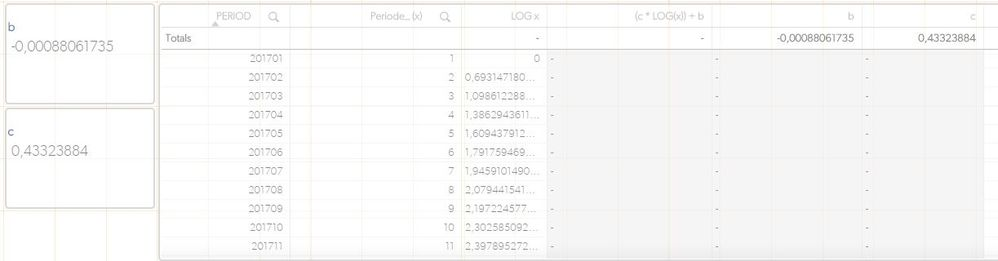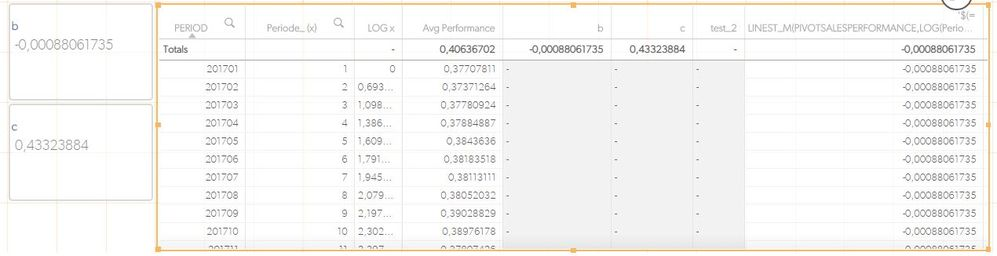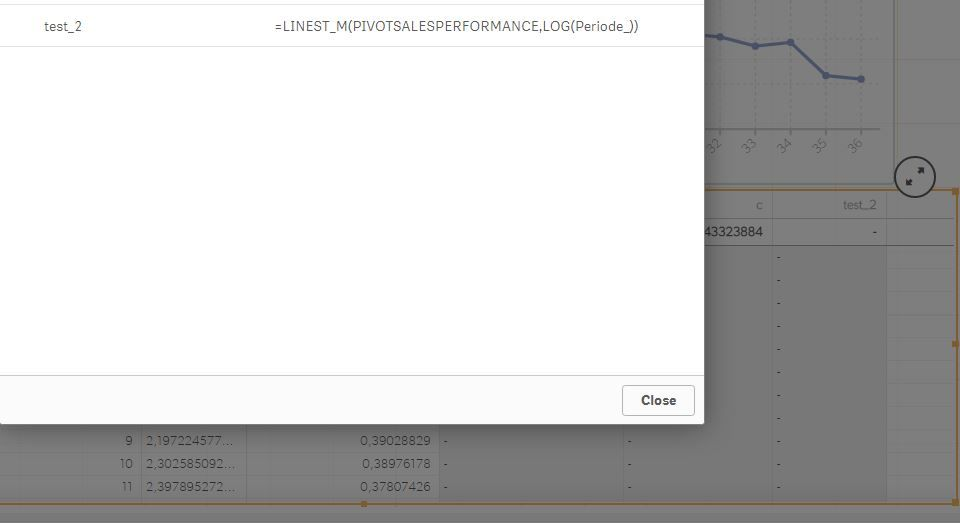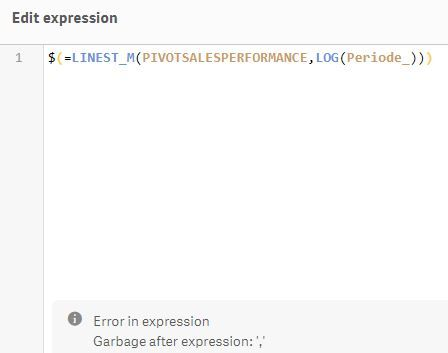# QlikView App Dev

Discussion Board for collaboration related to QlikView App Development.

cancel
Showing results for
Did you mean:Contributor III

## How do I solve this?

Hi,

Yesterday I also posted this in another section but it remains unanswered, probably the wrong section... The question I have is probably very simple but I am still new to Qlik. I have a table in which with a dimension I group. Then per group the calculation for log trendline should be made. I calculate the Log (x) and that is ok. But how do I get into this table the repeating values b & c that are calculated without the grouping in the table? It shows the results as a total but that does not help me. How is this done in Qlik?Thank you very much!

1 Solution

Accepted SolutionsContributor III
Author

You are a genius...10 RepliesMVP

HI, if b and c were variables they should be accesibles by all rows, maybe you are loading them as field values, not realted the the model, or at least not related to the data rows where period and x are.

You can try using variables for b and c, so you can use (\$(c)*LOG(x))+\$(b)

Or maybe using TOTAL to ignoer chart dimensions: (Only(TOTAL c)*LOG(x))+Only(TOTAL b)Creator

I believe use of Set parameters, more suitable here.

(\$(c)*LOG(x))+\$(b).Contributor III
Author

If I use this:

Only(TOTAL LINEST_M(PIVOTSALESPERFORMANCE,LOG(Periode_)))

It tells me that nested aggregation is not allowed

When using

\$(LINEST_M(PIVOTSALESPERFORMANCE,LOG(Periode_)))

There is no error but also no result.MVP

If that's one of the values calculated in a text box it can be a variable with value "=LINEST_M(PIVOTSALESPERFORMANCE,LOG(Periode_))"

And \$(VariableName) will return the calculated value.

Or also you can use \$(=LINEST_M(PIVOTSALESPERFORMANCE,LOG(Periode_)))Contributor III
Author

Hi, I will try. What I expect is:

The calculation for b = LINEST_M(PIVOTSALESPERFORMANCE,LOG(Periode_))
The calculation for c = LINEST_B(PIVOTSALESPERFORMANCE,LOG(Periode_))

What I expect, for example in the line where PERIOD = 201702 and periode_ = 2 is, using the next formula: y = (c * LOG(x)) + b

y = (0,43323884 * 0,69314718055995) -0,00088061735
y = 0,299417663Contributor III
Author

In both cases, it stays empty:What am I doing wrong?MVP

test_2 should not have the double quotes, I put them only to fix that the equal sign was part of the value of the variable

test_3 shouldn't be a variable, that's an example you can use directly on chart expressionContributor III
Author

OK, I expected that already... But if I change the variable, the issue maintains. If I use it as a straight as you mention I get a "garbage" error:MVP

Maybe it's beacuse it's returning a decimal value with comma, can you check adding single quotes to return as text and check the value?:

'\$(=LINE....)'Community Browser